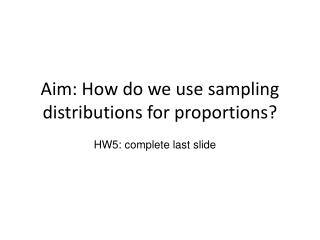DownloadDownload PresentationAim: How do we use sampling distributions for proportions?

# Aim: How do we use sampling distributions for proportions?

Download Presentation## Aim: How do we use sampling distributions for proportions?

- - - - - - - - - - - - - - - - - - - - - - - - - - - E N D - - - - - - - - - - - - - - - - - - - - - - - - - - -
##### Presentation Transcript

1. Aim: How do we use sampling distributions for proportions? HW5: complete last slide

2. Recap Definitions • Variable: a characteristic or attribute that can assume different values • Random Variable: a variable whose values are determined by chance • Discrete Variable: have a finite number of possible values or an infinite number of values tat can be counted • Continuous Variable: variables that can assume all values in the interval between any two given values - they are obtained from data that can be measured rather than counted

3. Statistical Inference • Statistical inference draws conclusions about a population or process on the basis of data • Data is summarized by statistics such as means, proportions, and slopes of regression lines • When data are produced by random sampling, a statistic is a random variable that obeys the laws of probability theory • Sampling distributions of statistics provides the link between probability and data

4. Sample Distribution • Shows how a statistics would vary in repeated data production. • It is a probability distribution that answers the question “What would happen if we did this many times?” • A statistic from a random sample or randomized experiment is a random variable. The probability of the statistics is its sample distribution.

5. Population Distribution • The population distribution of a variable is the distribution of its values for all members of the population. The population distribution is also the probability distribution of the variable when we choose one individual at random from the population.

6. Discrete Probability Distribution • Discrete Probability Distribution: consists of the values a random variable can assume and the corresponding probabilities of the values. The probabilities are determined theoretically or by observation.

7. Example • A sample survey asks 2000 college students whether they think that parents put too much pressure on their children. We would like to view the responses of these students as representative of a larger population of students who hold similar beliefs. That is, we will view the responses of the sampled students as an SRS from a population.

8. SRS • SRS = Stratified Random Sample • First divide the population into groups of similar individuals called strata • Then choose a separate SRS in each stratum and combine these SRSs to form the full sample

9. How do we read the example? • When there are only two possible outcomes for a random variable, we can summarize the results by giving the count for one of the possible outcomes. • We let n represent the sample size and we use X to represent the random variable that gives the count for the outcome of interest. • In our sample survey of college students, n = 2000 and X is the number of students who think that parents put too much pressure on their children. • Suppose X = 840. The random variable of interest is X and its value is 840.

10. Choosing the meaning of the random value X • In our example, we chose the random variable X to be the number of students who think that parents put too much pressure on their children. • We could have chosen X to be the number of students who do not think that parents put too much pressure on their children. • The choice is yours!

11. Interpreting Random Value X • To interpret the meaning of the random variable X in this setting, we need to know the sample size n. • The conclusion we would draw about student opinions in our survey would be quite different if we had observed X = 840 from a sample of size n = 1000.

12. Sample Proportion • When a random variable has two possible outcomes, we can use the sample proportion,as a summary. • The sample proportion of students surveyed who think that parents put too much pressure on their children is

13. Example • In a random sample of 150 seniors, 45 report taking transportation to school. • Give n, X and for this setting • Solution: n = 150 X = transportation 45 OR n = 150 X = no transportation 105

14. Class Work #2 • Worksheet

15. Homework • Explain what is wrong in each of the following scenarios. • If you toss a fair coin three times and a head appears each time, then the next toss is more likely to be a tail than a head. • If you toss a fair coin three times and a head appears each time, then the next toss is more likely to be a head than a tail. • Sample proportion is one of the parameters for a binomial distribution. • A poll of 1500 college students asked whether or not they had used the Internet to find a place to live sometime within the past year. There were 525 students who answered “Yes”; the other 975 answered “No.” • What is n? • Choose one of the two possible outcomes to define the random variable, X. Give a reason for your choice. • What is the value of X? • Find the sample proportion.